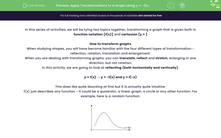# Apply Transformations to a Graph Using y = -f(x) and y = f(-x)

In this worksheet, students will practise applying the transformation y = -f(x) and y = f(-x) to a function defined as y = f(x)Key stage:  KS 4

Year:  GCSE

GCSE Subjects:   Maths

GCSE Boards:   AQA, Eduqas, OCR, Pearson Edexcel,

Curriculum topic:   Algebra, Graphs of Equations and Functions

Curriculum subtopic:   Graphs Transformation of Curves and Their Equations

Difficulty level:#### Worksheet Overview

In this series of activities, we will be tying two topics together, transforming a graph that is given both in function notation [f(x)] and cartesian [y = ]

How to transform graphs

When studying shapes, you will have become familiar with the four different types of transformation - reflection, rotation, translation and enlargement.

When you are dealing with transforming graphs, you can translate, reflect and stretch, enlarging in one direction, but not rotation.

In this activity, we are going to look at reflecting (both horizontally and vertically).

y = f(x) → y = -f(x) and y = f(-x)

This does like quite daunting at first but it is actually quite intuitive.

f(x) just describes any function - it could be a quadratic, a linear graph, a circle or any other function. For example, here is a random function:If we apply the transformation y = -f(x), every value of y becomes negative and the value of x remains the same. This will lead to a reflection in the x-axis.

If we apply the transformation y = f(-x), every value of x becomes negative and the value of y remains the same. This will lead to a reflection in the y-axis.How to apply this to a graph

Example:

You are given the function y = x2 - 4

Sketch the graph of y = 4 – x2

With this example, you have to rearrange the function y = 4 – x2 so that it is a transformation of the original function.

If we factorise out the value of -1, we will get y = 4 – x2 → y = -( x2 - 4)

From this, we can see that y = 4 – x2 is a reflection in the x-axis of y = x2 - 4Example:

You are given the function y = x2 - 3x - 4

Sketch the graph of y = x2 + 3x - 4

This one is a bit more tricky but you still have to rearrange the function y = x2 + 3x – 4 so that it is a transformation of the original function y = x2 - 3x – 4

This one has to be done by a process of elimination.

You should be able to eliminate all the other transformations, so we try to see if this is y = f(x) → y = f(-x)

y = (- x)2 + -3 (- x) – 4

y = x2 + 3x – 4

From this, we can see that y = x2 + 3x – 4 is a reflection in the y-axis of y = x2 - 3x – 4Time for some questions now.

### What is EdPlace?

We're your National Curriculum aligned online education content provider helping each child succeed in English, maths and science from year 1 to GCSE. With an EdPlace account you’ll be able to track and measure progress, helping each child achieve their best. We build confidence and attainment by personalising each child’s learning at a level that suits them.

Get started••••